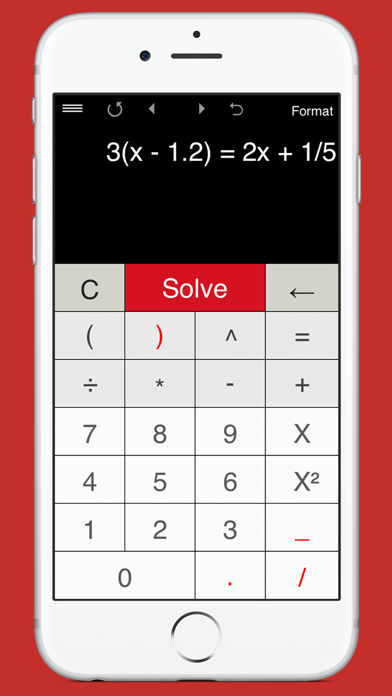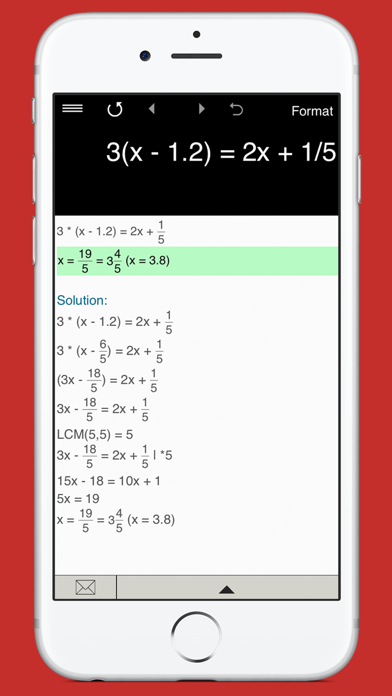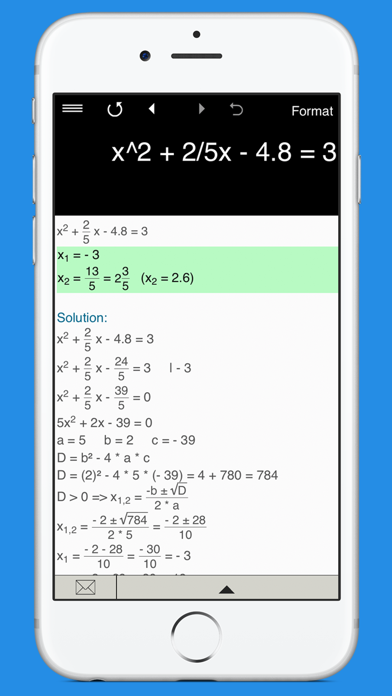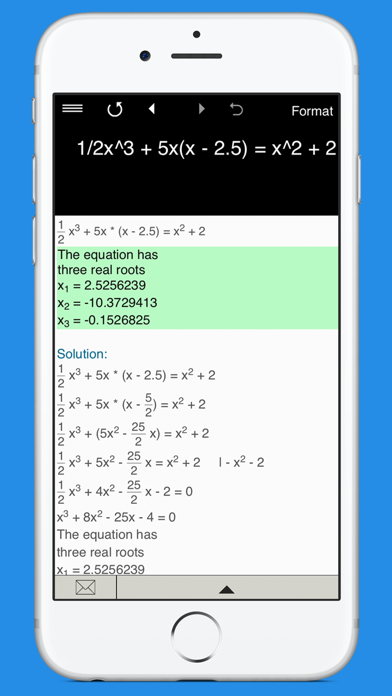## Equation Solvers - Lumos Educational App StorePrice -\$3.99

#### DESCRIPTION:

Equation Solver 4-in-1 is the ultimate app that helps you solve linear equations in one variable, quadratic equations with real and complex roots, cubic and quartic equations. It's intuitive and easy to use. Just type in any equation you want to solve and the equation calculator will show you the result. Everything you need to solve equations or check results! Features: • Linear Equation Solver. • Quadratic Equation Solver. • Cubic Equation Solver. • Quartic Equation Solver. • Solves equations with fractions and parentheses. Note: the app doesn’t yet support equations with a v

#### OVERVIEW:

Equation Solvers is a free educational mobile app By Intemodino Group s.r.o..It helps students practice the following standards .

This page not only allows students and teachers download Equation Solvers but also find engaging Sample Questions, Videos, Pins, Worksheets, Books related to the following topics.

Developer URL: https://intemodino.com

Software Version: 4.0.3

Category: Education

Release Date: 2012-11-11T06:29:34Z#### Are you the Developer?

Rate this App?
0

0 Ratings & 0 Reviews

5
0
0
4
0
0
3
0
0
2
0
0
1
0
0EdSearch WebSearch